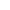###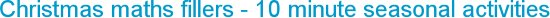Thursday, 24 November 2016
Christmas maths fillers are useful for those spare moments during these busy weeks in school. Here are a few short, one-off simple activities that can be done with only paper and crayons. They are interesting, fun maths tasks providing an opportunity for exploration and problem solving.

A printer wants to make rectangle advent calendars with 24 square doors.

How many different rectangle advent calendars can you make?

Draw rectangles with 24 sqaures on squared paper and write the numbers 1-24 on each square door.

Make other shapes with 24 squares. Can you make the shapes symmetrical?

How many pairs of doors can you find that total 25?
25th December is the date of Christmas day.

Can you find a set of 3 doors that total 25? How many sets of 3 doors total 25?

Investigate other sets of doors (4, 5, 6... doors) that total 25.
Rectangle advent calendars with square doors.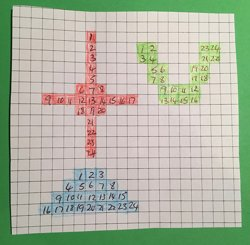• Christmas Alphabet

#### 1  2   3  4  5   6  7  8  9  10  11 12  13 14 15 16  17 18  19 20  21  22  23  24  25  26   A  B  C D  E  F  G  H  I   J   K   L   M  N   O   P  Q   R   S   T   U    V   W   X    Y   Z

Use the code to find Christmas decade words that total 10, 20, 30, 40 ...

T    U    R   K   E  Y    is a 'total 100' word
20+21+18+11+5+15

Can you find other 'total 100' words?

• Shapes in Stars

Draw triangles on isometric paper to make a hexagon. Extend the shape with a triangle on each side to make a 6-pointed star.

Triangle total

How many different size trangles can you see on the star?
How many triangles of each size can you count?
How many triangles are there altogether on this star?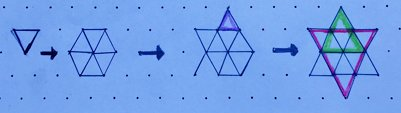Go on a rhombus hunt

Can you find a rhombus on the star?
Can you find the same shape in different sizes?
How many rhombuses of each size can you find? How many altogether?

Draw a rhombus, then draw another beside it. Can you draw the same star by adding one more rhombus each time?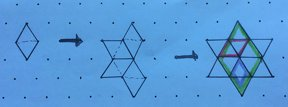Track down a trapezium

Can you see a trapezium on the star?
Can you find different sizes?
How many can you find in total?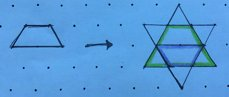Related articles

Christmas maths problem solving
The 12 days of Christmas investigations - how many presents altogether?

How many ways can a star be drawn using 2D shapes?
Activities for both KS1 and KS2 using 2D shapes

How much paper is needed to make a parcel?
Make different cuboids with 36 interlocking cubes, how much paper is needed for each one?

Make a paper star for Christmas
Step by step instructions to fold a star with lots of maths

Fibonnaci numbers on Christmas cones

Showing how to count the spirals on a cone - what are the numbers you find?
Website design by SiteBuilder Bespoke